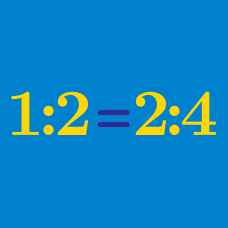Everyday Math

# Ratio, Rate and Proportion - Problem Solving

What is the value of $x$ that satisfies the proportional equation $(5x-13):(6x-8)=4:5?$

If Calvin paid $\5$ for $7$ pencils, how much would he pay for $56$ pencils?

If a map represents the actual distance with a ratio of $1 \text{ cm}$ to $10 \text{ km},$ then what is the actual size for $7 \text{ cm}^2$ on the map?

Charlie rides his bicycle down a hill at 30 kilometers per hour. Then he turns around and rides back up the hill at 6 kilometers per hour to his starting point. What is Charlie's average speed for the whole trip down and back up the hill?

The ratio of the base to the height of a right triangle is $3:5.$ If the area of this right triangle is $30,$ what is the height of the right triangle?

×